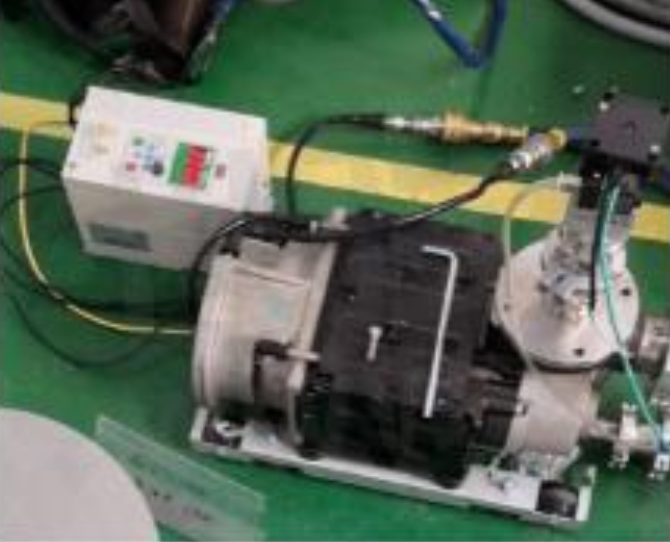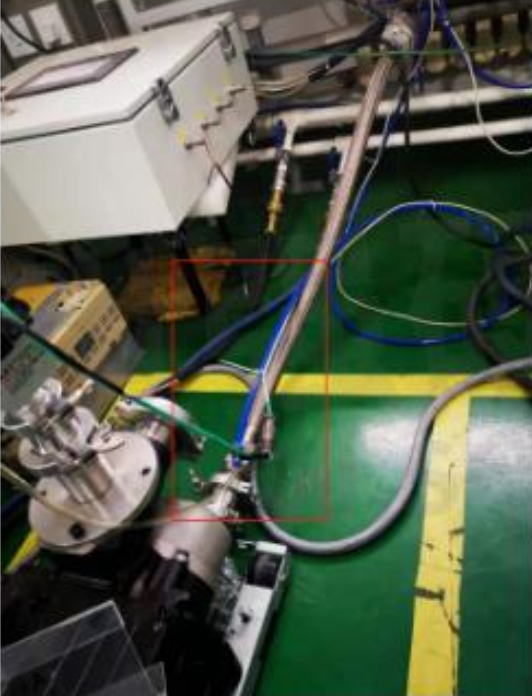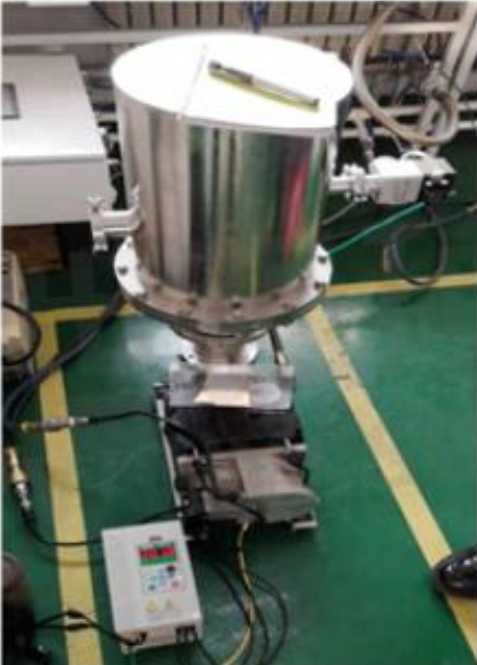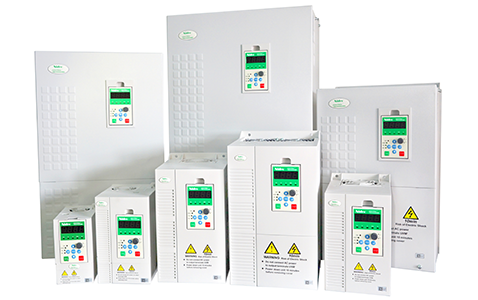## 尼得科CT高功能矢量驱动器NE200应用在真空泵永磁电机上，抗负载突变能力强，最高频率可达3300Hz1、恢复出厂设置：FP.02=2（恢复出厂值）

2、参数设置

F0.01=1         （SVC）

F0.10=300Hz （基本频率，同电机额定频率）

F0.11=350Hz （最大频率）

F0.12=350Hz （上限频率）

F0.14=155V   （最大输出电压）

F0.19=10s     （加速时间）

F5.00=2         （电机类型：选择永磁电机）

F5.01=4         （电机级数）

F5.03=8.3A   （额定电流）

F5.04=9000  （额定转速）

FP.05=0        （G型机）

3、电机自学习

F5.10=2（旋转自整定）

F5.11=454mΩ（定子电阻）

F5.12=2.68mH（Ld电感）

F5.13=3.10mH（Lq电感）

F5.14=8.9V     （反向电动势）

4、注意：

NE系列是最高频率对应的反向电动势电压，M系列是1000rpm对应的反向电动势电压。Source: <https://www.sekorm.com/news/77177550.html>